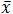# Chapter 4: Probability Distributions

Answers for all ‘Test Yourself’ questions from the book to check your performance and widen your overall understanding of the contents.

1.
1. The uniform distribution, because there two and only two possibilities, and each of them is equally probable.
2. The Poisson distribution, because employee absences are counts of relatively unusual occurrences.
3. The normal distribution, because psychological data tends to fall in a normal distribution (values vary randomly around a population mean).
2. To answer this question, remember to carry out all calculations to 6 decimal places, only rounding to 2 at your final answer.
1. 4.12 * 2.64 = 10.8768 = 10.88
2. (7.1 / 2.31) / 4.1 = (3.073593) / 4.1 = 0.749657 = 0.75
3. 2.52 + 3.332 = 6.25 + 11.0889 = 17.3389 = 17.34
3. If= 5 and s = 2, calculate X for the following z scores:
1. X = 8
2. X = -1
3. X = 9.5
4. To answer this question, reference the overall picture of the z-distribution found on p. ##
1. .8385
2. .16
3. .975